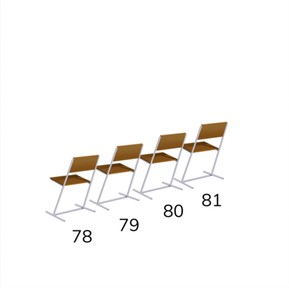Comparing and ordering numbers to 100

# Comparing and ordering numbers to 100

I can compare numbers from 1 to 100 and put them in the correct order.

No account needed.8,000 schools use Gynzy92,000 teachers use Gynzy1,600,000 students use Gynzy

## General

Students learn to compare the numbers from 1 to 100 and are able to set them in numerical order. They can determine when a number is more or less than a given number.

-1.NBT.B.3

## Relevance

Discuss with students why it is useful to be able to compare numbers and set them in numerical order. Say that you can use this to determine where your chair should be at a concert.

## Introduction

The interactive whiteboard shows a selection of problems where students need to figure out where a number belongs on the number line.

## Development

Show students a number line on the interactive whiteboard and explain that it shows the value of the numbers. The value increases from left to right and numbers on the left are smaller than the numbers on the right. They go from least to most. Set a few numbers on the number line with the class and and show how they go from least to most and it is represented by their position on the number line. The number that comes first on the number line is the smallest, or least, and the last number you set on the number line is the biggest, or most. As a class set a few numbers in order. Tell students that they can use the number line as a tool to set the numbers in the correct order.

To check that students understand comparing and ordering numbers to 100 you can ask the following questions/give the following exercises:
- When is a number more or less than another number?
- How do you know that a number is more or less than another?
- Which number is more than 39? How do you know?
Write a number that is less than 45.
- Write a number that is more than 72.

## Guided practice

Students first practice with the terms more, less, most and least with visual support. Next they use these terms in story form and also in numerical form to set numbers in order.

## Closing

Discuss with students that it is important to be able to compare numbers and set them in the right order, for example in situations like a concert when you are looking for your seat number. Check that students know that they can use a number line as support and they know how to set the numbers in order from least to most. To check this set students in groups. Everyone must write a number from 1 to 100. They must then set these numbers in order from least to most.

## Teaching tips

Students who have difficulty comparing and ordering numbers can use the number line as support. By placing numbers on the number line, they can see which number is least and most, and follow the order in which they should be set.

### The online teaching platform for interactive whiteboards and displays in schools

• Save time building lessons

• Manage the classroom more efficiently

• Increase student engagement